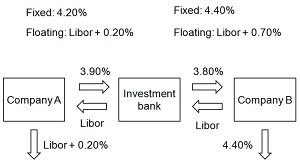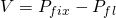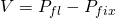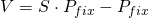# Swap valuation

An interest rate swap is an agreement in which 2 parties agree to periodically exchange cash flows over a certain period.The amount of money exchanged depends on the principal amount, the floating and fixed rate. Swaps can both be for hedging and speculating as well as lowering the funding cost for a company or country. This is shown in the figure below in which both parties can lower their funding cost by 0.10%. company A now borrows at fixed rate of 4.10% instead of 4.20%, company borrows at floating Libor + 0.60% instead of Libor + 0.70% as shown in the figure below.# Interest rate swap valuation

The valuation of an interest rate swap can be approached through bond combinations. In case an investor receives a fixed rate and pays floating, the value of the swap, V, is just the difference between the value of a fixed rate bond,Pfix, and a floating rate bond, Pfl. See sections fixed rate bond valuation and floating rate bond valuation to value these bonds. Depending on whether you are the fixed rate payer or receiver, the following formula calculates the swap value to you. In case you receive the fixe rate, the first equation is applicable, otherwise the second is applicable.## Currency swap valuation

The valuation of a currency swap is very similar to those of an interest rate swap. The difference lies in the fact that 1 cash flow has to be converted to the other currency based on the spot fx price, S, in which the swap is priced. Currency swaps can be fixed-for-fixed, fixed-for-floating or floating-for-floating.## Summary

Interest rates swaps are used to lower funding costs, speculate or hedge future cash flows. The value of an interest rate swaps equals the difference of an interest rate paying and receiving bond.

### Swap valuation Excel implementation

Need to have more insights? Download our free excel file: swap valuation.